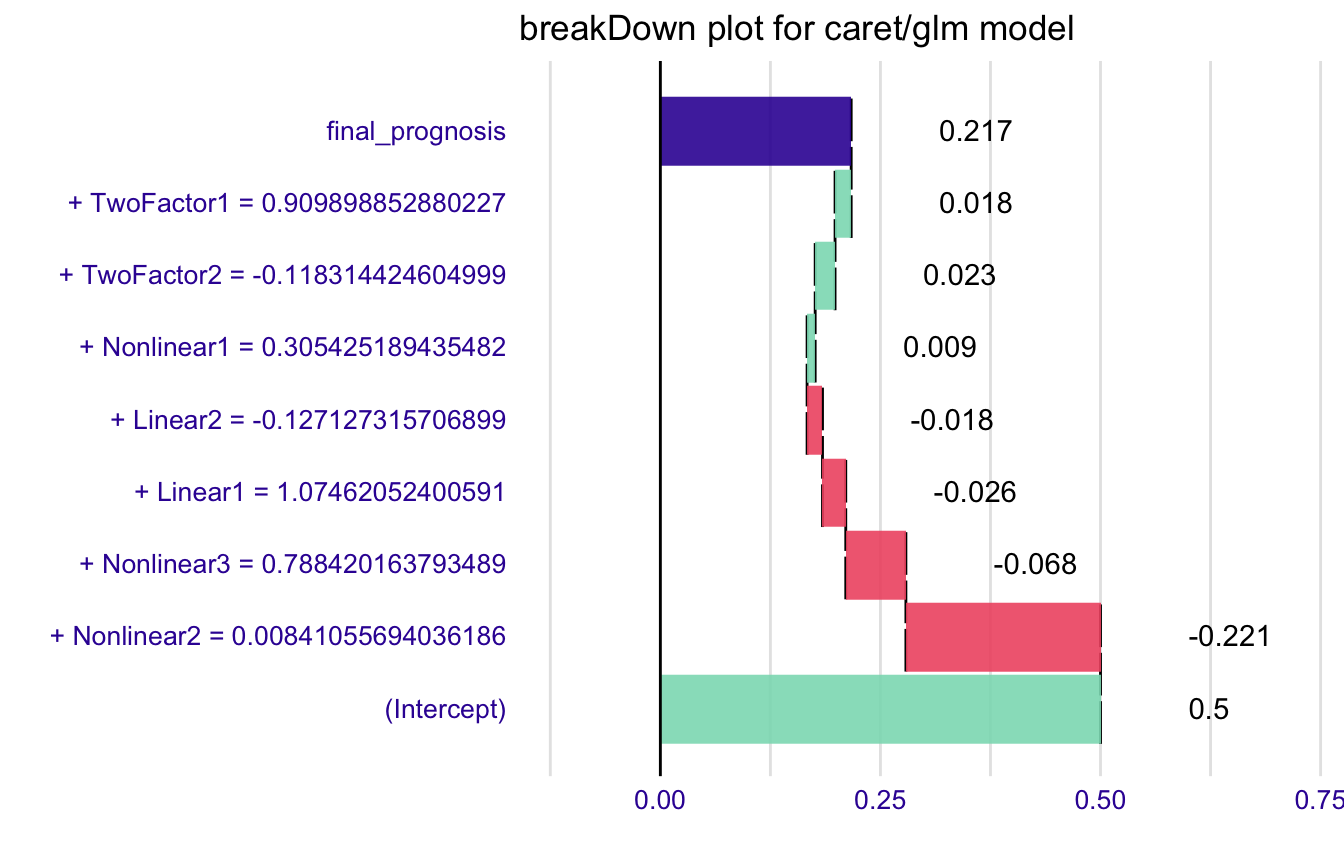This example demonstrates how to use the breakDown package for models created with the caret package.

First we will generate some data.

library(caret)

set.seed(2)
training <- twoClassSim(50, linearVars = 2)
trainX <- training[, -ncol(training)]
trainY <- training\$Class

#>   TwoFactor1 TwoFactor2    Linear1    Linear2 Nonlinear1 Nonlinear2
#> 1 -0.6561702 -1.6480450  1.0744594  0.9758906  0.2342843  0.6805653
#> 2 -0.9849973  1.4598834  0.2605978 -0.1694232  0.1381283  0.7460168
#> 3  2.3722541  1.7069944 -0.3142720  0.7221918 -0.6920591  0.4642024
#> 4 -2.2067173 -0.6972704 -0.7496301 -0.8444186 -0.9303336  0.1374181
#> 5  0.5166671 -0.7228376 -0.8621983  1.2772937  0.9959069  0.8143796
#> 6  1.3331262 -0.9929323  2.0480403 -1.3431105  0.6711474  0.8321613
#>   Nonlinear3  Class
#> 1  0.6920055 Class1
#> 2  0.5599569 Class2
#> 3  0.3426912 Class1
#> 4  0.2344975 Class2
#> 5  0.4296028 Class1
#> 6  0.7367007 Class1

Now we are ready to train a model. Let’s train a glm model with caret.

cctrl1 <- trainControl(method = "cv", number = 3, returnResamp = "all",
classProbs = TRUE,
summaryFunction = twoClassSummary)

test_class_cv_model <- train(trainX, trainY,
method = "glm",
trControl = cctrl1,
metric = "ROC",
preProc = c("center", "scale"))
test_class_cv_model
#> Generalized Linear Model
#>
#> 50 samples
#>  7 predictor
#>  2 classes: 'Class1', 'Class2'
#>
#> Pre-processing: centered (7), scaled (7)
#> Resampling: Cross-Validated (3 fold)
#> Summary of sample sizes: 34, 32, 34
#> Resampling results:
#>
#>   ROC        Sens       Spec
#>   0.7420267  0.7962963  0.712963

To use breakDown we need a function that will calculate scores/predictions for a single observation. By default the predict() function returns predicted class.

So we are adding type = "prob" argument to get scores. And since there will be two scores for each observarion we need to extract one of them.

predict.fun <- function(model, x) predict(model, x, type = "prob")[,1]
testing <- twoClassSim(10, linearVars = 2)
predict.fun(test_class_cv_model, testing[1,])
#>  0.2167808

Now we are ready to call the broken() function.

library("breakDown")
explain_2 <- broken(test_class_cv_model, testing[1,], data = trainX, predict.function = predict.fun)
explain_2
#>                                    contribution
#> (Intercept)                               0.500
#> + Nonlinear2 = 0.00841055694036186       -0.221
#> + Nonlinear3 = 0.788420163793489         -0.068
#> + Linear1 = 1.07462052400591             -0.026
#> + Linear2 = -0.127127315706899           -0.018
#> + Nonlinear1 = 0.305425189435482          0.009
#> + TwoFactor2 = -0.118314424604999         0.023
#> + TwoFactor1 = 0.909898852880227          0.018
#> final_prognosis                           0.217
#> baseline:  0

And plot it.

library(ggplot2)
plot(explain_2) + ggtitle("breakDown plot for caret/glm model")